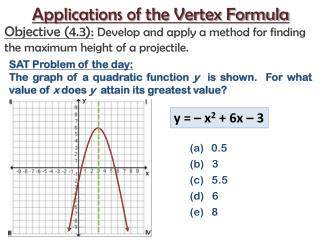DownloadDownload PresentationSAT Problem of the day:

# SAT Problem of the day:

Download Presentation## SAT Problem of the day:

- - - - - - - - - - - - - - - - - - - - - - - - - - - E N D - - - - - - - - - - - - - - - - - - - - - - - - - - -
##### Presentation Transcript

1. Applications of the Vertex Formula Objective (4.3):Develop and apply a method for finding the maximum height of a projectile. SAT Problem of the day: The graph of a quadratic function y is shown. For what value of x does y attain its greatest value? y = – x2 + 6x – 3 • 0.5 • (b) 3 • (c) 5.5 • (d) 6 • (e) 8

2. Vocabulary • re-visited • A projectile is an object in which the only force acting upon it is gravity. • A parabola is the graph of a quadratic function.

3. Which of the following would NOT be considered aprojectile? • (a)Dropping a pumpkin off of a building. • (b)A plane taking off and then landing. • (c)Driving a golf ball. • (d)A person jumping on trampoline.

4. Thevertexof a parabola is either the lowest point on the graph or the highest point on the graph. Vertex Vertex • book page 276

5. When a parabola opens up and the vertex • is the lowest point the y-coordinate of the • vertex is the minimum. maximum minimum • When a parabola opens down and the • vertex is the highest point the y-coordinate • of the vertex is the maximum. • book page 277

6. When a parabola opens up its lowest point is known as the: • (a)minimum • (b)maximum • (c)vertex • (d)a & c • (e)b & c • (f)all of the above

7. To help identify the vertex of a quadratic function we can use the following formula: – b 2a x =

8. Where do we see quadratic functions in our everyday lives? http://www.polleverywhere.com/free_text_polls/NzkwNjI3MjIw pollanywhere.com

9. Where do we see quadratic functions in real life?

10. What component do we often neglect when applying formulas for projectile motion? • (a)initial height • (b)initial velocity • (c)height • (d)air resistance • (e)velocity

11. Consider a firework display.

12. Collins Writing Type I: When a projectile is released into the air a number of factors come into play including initial height, maximum height, time, and velocity. If you were designing a firework display why do you think each of these factors would be important? Time: 90 seconds Length: 3 Lines http://www.online-stopwatch.com/large-stopwatch/

13. When a projectile is released into the air, what types of factors come into play? h = –16t2 + v0t + h0 h0 Initial Height Term used to represent the earth’s gravity. h Height t Time v0 Initial Velocity (or speed)

14. The path of a firework can be modeled using a quadratic function h = –16t2 + v0t + h0 – b 2a t= We can use the vertex formula to determine the time it takes for a firework to explode, and the maximum height that it reaches.

15. On July 4th Ocean City has a firework display. The fireworks are ignited from the football field with an initial velocity of 96 feet per second. How long does it take for the fireworks to reach their maximum height? What is the maximum height reached by the fireworks? the football field initial velocity of 96 How long h = –16t2 + v0t + h0 maximum height – b 2a t=

16. A professional pyro-technician shoots fireworks vertically into the air off of a building that is 80 feet tall. The initial velocity of the firework is 64 feet per second. When will the fireworks reach their maximum height? What is the maximum height reached by the fireworks? 80 feet tall initial velocity 64 feet per second When h = –16t2 + v0t + h0 maximum height – b 2a t=

17. Textbook page 313 Numbers 49 & 50

18. A baseball is thrown upward with an initial velocity of 48 feet per second from 6 feet above the ground. Determine the maximum height of the ball. initial velocity of 48 feet per second 6 feet above the ground maximum height h = –16t2 + v0t + h0 – b 2a t=

19. EXIT TICKET – b 2a t= h = –16t2 + v0t + h0